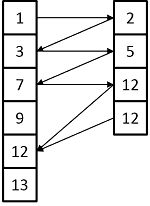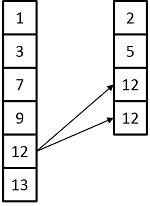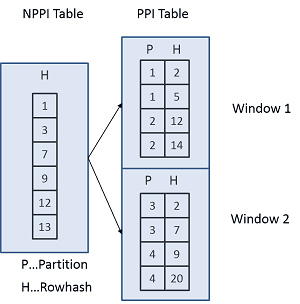# Teradata Sliding Window Merge Join: A Detailed Explanation

Roland Wenzlofsky

April 15, 2015

## Introduction to the Teradata Sliding Window Merge Join

DWH Pro thoroughly analyzes the Teradata sliding window merge join, which has limited available information. Our extensive research provides valuable insights into this process.

To understand the Teradata sliding window merge join, we must first review the join algorithms used in the conventional merge join. This will provide a foundation for the join.

``````Requirements:

The rows to be joined have to be located on the same AMP
Both spools have to be sorted by the ROWID calculated over the join column(s)

Possible Join Preparations required:

Re-Distribution of one or both spools by ROWHASH or
Duplication of the smaller spool to all AMPs
Sorting of one or both spools by the ROWID

Teradata can use two different algorithms for the Merge Join:
1. The Fast Path Algorithm
The comparison occurs alternating from both sides, starting with the left table. The algorithm tries to join rows with matching rowhash. If there is no match, the pointer is positioned on the row with the next highest rowhash value, and the comparison continues until all rows have been compared.
This method is used if the left and right table are full table scanned:
2. The Slow Path Algorithm
This algorithm reads each row from the left table and matches it against rows with the same rowhash from the right table.
This method is used if the left table is accessed via an index:

Traditional Merge Joins have a significant advantage over other join types, which don't require both tables to be sorted by rowhash of the join columns:
Each data block of both tables is accessed once (the algorithm slides down on each of the rowhash sorted tables). It is less sensitive to the size of the available FSG cache.
The Teradata Sliding Window Merge Join
The sliding window merge join is an advancement of the traditional merge join. After introducing the feature of row partitioning, it was required to find an algorithm that allows joining a row partitioned table (PPI table) with

Another PPI table has different partition characteristics
A Non-partitioned table

The optimizer can change a PPI table into an NPPI table and vice versa. A traditional merge join can follow this step. Nevertheless, to avoid this join preparation step, a sliding window merge join can be executed without the restructuring of tables.
As opposed to non-partitioned tables (NPPI tables), which are only sorted by rowhash, PPI tables are sorted on each AMP by two levels:
1. Each row is placed into its assigned row partition.2. Within each row partition, the rows are sorted by rowhash (the same way the rows of an NPPI table are stored).
The Sliding Window Merge Join allows joining directly.

An NPPI table with a PPI table or
Two PPI tables with different partitioning

Directly means without changing a PPI table into an NPPI table or the other way around.
To understand the sliding window join process, one has to remember how PPI table rows are stored:
Data rows are sorted by rowhash within the data blocks, but several row partitions can hold the same rowhash value!
The easiest way to merge joining an NPPI table against a PPI table seems to be by joining the NPPI table against each PPI table partition. This approach would be a reasonable solution because the NPPI table and each partition of the PPI table is sorted by rowhash, allowing them to execute a binary search within each partition's data blocks.
Nevertheless, for performance reasons, Teradata implements a slightly different but faster algorithm:
The AMP reads the first data block from the NPPI table and one data block per partition from the PPI table. The rows are joined (binary search), and the algorithm moves down both tables by reading data block after data block from both tables.
The data block of the NPPI tables stays in the FSG cache as long as data blocks from any partition of the PPI tables can be matched. If no more rows can be matched, the following data block of the NPPI tables is moved into the FSG cache, and the above-described process is repeated until the last data blocks of each table and partition are reached.
This process requires each table's data block to be touched exactly once (similar to a traditional merge join).
This process could theoretically result in a similar join performance as we can achieve with a traditional merge join. Still, one restriction degrades performance: The available FSG cache memory.
If the FSG cache is not enough to hold at least one data block from each PPI table partition, the optimizer must split the join process into so-called windows.
Assume, for example, that the PPI tables consist of 4 partitions, but there is only space in the FSG cache for the first data block of  2 partitions. In this case, the process would define two windows, each one consisting of 2 partitions:

Join the NPPI table against the first two partitions
Join  the NPPI table against the remaining two partitions

As a result, the NPPI table must be read twice, and the join becomes costly. The more windows are needed, the more expensive the join becomes. If the NPPI table is small enough, caching effects could decrease the negative performance impact of having several windows.
The sliding window merge join has a similar performance pattern like a traditional merge join if most of the partitions can be eliminated before a join takes place. The best-case scenario is when there is sufficient FSG cache available to join all partitions at once. This kind of setup is called a single-window merge join.
The join of two PPI tables with different partitioning is implemented similarly to the join between an NPPI and a PPI table:
The left table and the right table rows are split into windows (each window containing a part of the row partitions), and each window from the left table is joined to each window from the right table.
Here is an example: If the join needs to create five windows from the left PPI table and two windows from the right PPI table, this will result in a product join of 5*2 windows:
A sliding window merge join is a product joining each window of the left table with each right table window. The join process between 2 windows is similar to a traditional merge join process with a binary search on both tables.

``````•Agilan says:

How we could avoid sliding window merge join when it impacts the Join operation in terms of performance?